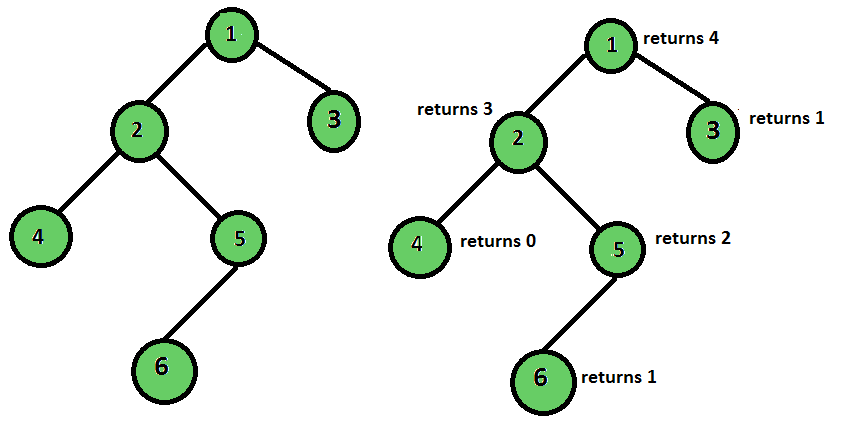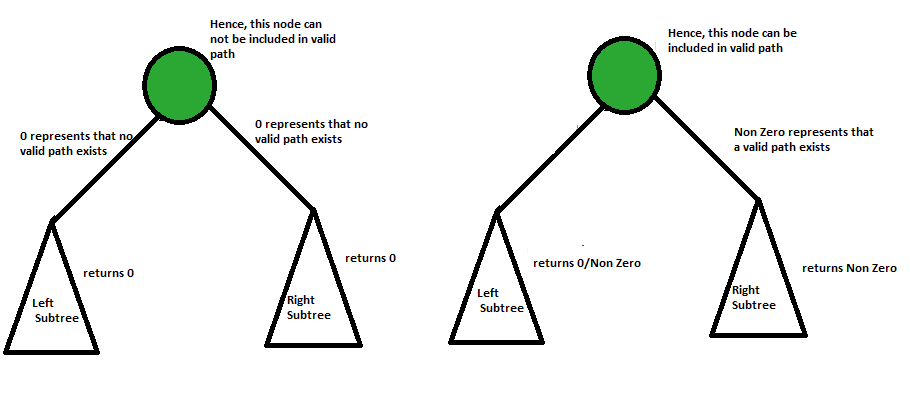# Height of binary tree considering even level leaves only

Find the height of the binary tree given that only the nodes on the even levels are considered as the valid leaf nodes.
The height of a binary tree is the number of edges between the tree’s root and its furthest leaf. But what if we bring a twist and change the definition of a leaf node. Let us define a valid leaf node as the node that has no children and is at an even level (considering root node as an odd level node).Output :Height of tree is 4

## Recommended: Please try your approach on {IDE} first, before moving on to the solution.

Solution : The approach to this problem is slightly different from the normal height finding approach. In the return step, we check if the node is a valid root node or not. If it is valid, return 1, else we return 0. Now in the recursive step- if the left and the right sub-tree both yield 0, the current node yields 0 too, because in that case there is no path from current node to a valid leaf node. But in case at least one of the values returned by the children is non-zero, it means the leaf node on that path is a valid leaf node, and hence that path can contribute to the final result, so we return max of the values returned + 1 for the current node.## C++

 `/* Program to find height of the tree considering ` `   ``only even level leaves. */` `#include ` `using` `namespace` `std; ` ` `  `/* A binary tree node has data, pointer to ` `   ``left child and a pointer to right child */` `struct` `Node { ` `    ``int` `data; ` `    ``struct` `Node* left; ` `    ``struct` `Node* right; ` `}; ` ` `  `int` `heightOfTreeUtil(Node* root, ``bool` `isEven) ` `{ ` `    ``// Base Case ` `    ``if` `(!root) ` `        ``return` `0; ` ` `  `    ``if` `(!root->left && !root->right) { ` `        ``if` `(isEven) ` `            ``return` `1; ` `        ``else` `            ``return` `0; ` `    ``} ` ` `  `    ``/*left stores the result of left subtree, ` `      ``and right stores the result of right subtree*/` `    ``int` `left = heightOfTreeUtil(root->left, !isEven); ` `    ``int` `right = heightOfTreeUtil(root->right, !isEven); ` ` `  `    ``/*If both left and right returns 0, it means ` `      ``there is no valid path till leaf node*/` `    ``if` `(left == 0 && right == 0) ` `        ``return` `0; ` ` `  `    ``return` `(1 + max(left, right)); ` `} ` ` `  `/* Helper function that allocates a new node with the ` `   ``given data and NULL left and right pointers. */` `struct` `Node* newNode(``int` `data) ` `{ ` `    ``struct` `Node* node =  ` `              ``(``struct` `Node*)``malloc``(``sizeof``(``struct` `Node)); ` `    ``node->data = data; ` `    ``node->left = NULL; ` `    ``node->right = NULL; ` ` `  `    ``return` `(node); ` `} ` ` `  `int` `heightOfTree(Node* root) ` `{ ` `    ``return` `heightOfTreeUtil(root, ``false``); ` `} ` ` `  `/* Driver program to test above functions*/` `int` `main() ` `{ ` `    ``// Let us create binary tree shown in above diagram ` `    ``struct` `Node* root = newNode(1); ` ` `  `    ``root->left = newNode(2); ` `    ``root->right = newNode(3); ` `    ``root->left->left = newNode(4); ` `    ``root->left->right = newNode(5); ` `    ``root->left->right->left = newNode(6); ` `    ``cout << ``"Height of tree is "` `<< heightOfTree(root); ` `    ``return` `0; ` `} `

## Java

 `/* Java Program to find height of the tree considering  ` `only even level leaves. */` `class` `GfG { ` ` `  `/* A binary tree node has data, pointer to  ` `left child and a pointer to right child */` `static` `class` `Node {  ` `    ``int` `data;  ` `    ``Node left;  ` ` ``Node right;  ` `} ` ` `  `static` `int` `heightOfTreeUtil(Node root, ``boolean` `isEven)  ` `{  ` `    ``// Base Case  ` `    ``if` `(root == ``null``)  ` `        ``return` `0``;  ` ` `  `    ``if` `(root.left == ``null` `&& root.right == ``null``) {  ` `        ``if` `(isEven == ``true``)  ` `            ``return` `1``;  ` `        ``else` `            ``return` `0``;  ` `    ``}  ` ` `  `    ``/*left stores the result of left subtree,  ` `    ``and right stores the result of right subtree*/` `    ``int` `left = heightOfTreeUtil(root.left, !isEven);  ` `    ``int` `right = heightOfTreeUtil(root.right, !isEven);  ` ` `  `    ``/*If both left and right returns 0, it means  ` `    ``there is no valid path till leaf node*/` `    ``if` `(left == ``0` `&& right == ``0``)  ` `        ``return` `0``;  ` ` `  `    ``return` `(``1` `+ Math.max(left, right));  ` `}  ` ` `  `/* Helper function that allocates a new node with the  ` `given data and NULL left and right pointers. */` `static` `Node newNode(``int` `data)  ` `{  ` `    ``Node node = ``new` `Node();  ` `    ``node.data = data;  ` `    ``node.left = ``null``;  ` `    ``node.right = ``null``;  ` ` `  `    ``return` `(node);  ` `}  ` ` `  `static` `int` `heightOfTree(Node root)  ` `{  ` `    ``return` `heightOfTreeUtil(root, ``false``);  ` `}  ` ` `  `/* Driver program to test above functions*/` `public` `static` `void` `main(String[] args)  ` `{  ` `    ``// Let us create binary tree shown in above diagram  ` `    ``Node root = newNode(``1``);  ` ` `  `    ``root.left = newNode(``2``);  ` `    ``root.right = newNode(``3``);  ` `    ``root.left.left = newNode(``4``);  ` `    ``root.left.right = newNode(``5``);  ` `    ``root.left.right.left = newNode(``6``);  ` `    ``System.out.println(``"Height of tree is "` `+ heightOfTree(root));  ` `} ` `}  `

## Python3

 `# Program to find height of the tree considering  ` `# only even level leaves. ` ` `  `# Helper class that allocates a new node with the  ` `# given data and None left and right pointers.  ` `class` `newNode: ` `    ``def` `__init__(``self``, data): ` `        ``self``.data ``=` `data  ` `        ``self``.left ``=` `None` `        ``self``.right ``=` `None` ` `  `def` `heightOfTreeUtil(root, isEven): ` `     `  `    ``# Base Case  ` `    ``if` `(``not` `root):  ` `        ``return` `0` ` `  `    ``if` `(``not` `root.left ``and` `not` `root.right):  ` `        ``if` `(isEven):  ` `            ``return` `1` `        ``else``: ` `            ``return` `0` ` `  `    ``# left stores the result of left subtree,  ` `    ``# and right stores the result of right subtree ` `    ``left ``=` `heightOfTreeUtil(root.left, ``not` `isEven)  ` `    ``right ``=` `heightOfTreeUtil(root.right, ``not` `isEven)  ` ` `  `    ``#If both left and right returns 0, it means  ` `    ``# there is no valid path till leaf node ` `    ``if` `(left ``=``=` `0` `and` `right ``=``=` `0``): ` `        ``return` `0` ` `  `    ``return` `(``1` `+` `max``(left, right)) ` ` `  `def` `heightOfTree(root): ` `    ``return` `heightOfTreeUtil(root, ``False``) ` ` `  `# Driver Code ` `if` `__name__ ``=``=` `'__main__'``:  ` `     `  `    ``# Let us create binary tree shown ` `    ``# in above diagram  ` `    ``root ``=` `newNode(``1``)  ` ` `  `    ``root.left ``=` `newNode(``2``)  ` `    ``root.right ``=` `newNode(``3``)  ` `    ``root.left.left ``=` `newNode(``4``)  ` `    ``root.left.right ``=` `newNode(``5``)  ` `    ``root.left.right.left ``=` `newNode(``6``)  ` `    ``print``(``"Height of tree is"``, ` `           ``heightOfTree(root)) ` ` `  `# This code is contributed by PranchalK `

## C#

 `/* C# Program to find height of the tree considering  ` `only even level leaves. */` `using` `System; ` ` `  `class` `GfG ` `{  ` ` `  `    ``/* A binary tree node has data, pointer to  ` `    ``left child and a pointer to right child */` `    ``class` `Node ` `    ``{  ` `        ``public` `int` `data;  ` `        ``public` `Node left;  ` `        ``public` `Node right;  ` `    ``}  ` ` `  `    ``static` `int` `heightOfTreeUtil(Node root, ` `                               ``bool` `isEven)  ` `    ``{  ` `        ``// Base Case  ` `        ``if` `(root == ``null``)  ` `            ``return` `0;  ` ` `  `        ``if` `(root.left == ``null` `&&  ` `            ``root.right == ``null``) ` `        ``{  ` `            ``if` `(isEven == ``true``)  ` `                ``return` `1;  ` `            ``else` `                ``return` `0;  ` `        ``}  ` ` `  `        ``/*left stores the result of left subtree,  ` `        ``and right stores the result of right subtree*/` `        ``int` `left = heightOfTreeUtil(root.left, !isEven);  ` `        ``int` `right = heightOfTreeUtil(root.right, !isEven);  ` ` `  `        ``/*If both left and right returns 0, it means  ` `        ``there is no valid path till leaf node*/` `        ``if` `(left == 0 && right == 0)  ` `            ``return` `0;  ` ` `  `        ``return` `(1 + Math.Max(left, right));  ` `    ``}  ` ` `  `    ``/* Helper function that allocates a new node with the  ` `    ``given data and NULL left and right pointers. */` `    ``static` `Node newNode(``int` `data)  ` `    ``{  ` `        ``Node node = ``new` `Node();  ` `        ``node.data = data;  ` `        ``node.left = ``null``;  ` `        ``node.right = ``null``;  ` ` `  `        ``return` `(node);  ` `    ``}  ` ` `  `    ``static` `int` `heightOfTree(Node root)  ` `    ``{  ` `        ``return` `heightOfTreeUtil(root, ``false``);  ` `    ``}  ` ` `  `    ``/* Driver code*/` `    ``public` `static` `void` `Main(String[] args)  ` `    ``{  ` `        ``// Let us create binary tree ` `        ``// shown in above diagram  ` `        ``Node root = newNode(1);  ` ` `  `        ``root.left = newNode(2);  ` `        ``root.right = newNode(3);  ` `        ``root.left.left = newNode(4);  ` `        ``root.left.right = newNode(5);  ` `        ``root.left.right.left = newNode(6);  ` `        ``Console.WriteLine(``"Height of tree is "` `+ ` `                            ``heightOfTree(root));  ` `    ``}  ` `}  ` ` `  `/* This code is contributed by Rajput-Ji*/`

Output:

```Height of tree is 4
```

Time Complexity:O(n) where n is number of nodes in given binary tree.

My Personal Notes arrow_drop_upCheck out this Author's contributed articles.

If you like GeeksforGeeks and would like to contribute, you can also write an article using contribute.geeksforgeeks.org or mail your article to contribute@geeksforgeeks.org. See your article appearing on the GeeksforGeeks main page and help other Geeks.

Please Improve this article if you find anything incorrect by clicking on the "Improve Article" button below.

Article Tags :
Practice Tags :

Be the First to upvote.

Please write to us at contribute@geeksforgeeks.org to report any issue with the above content.